Density activities Substances sink when placed in liquids that are less dense. Oil will float on top of a layer of water because it is less dense than water. This difference in density can be used to create toys such as those pictured on the right. Click to see how to make one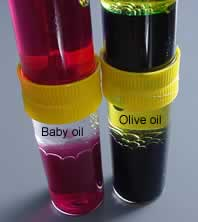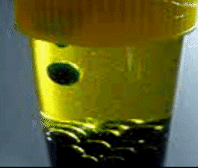A simple lava toy can be constructed using differences in density between oil and water. Click to see how to construct a simple lava toy.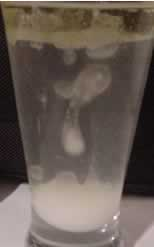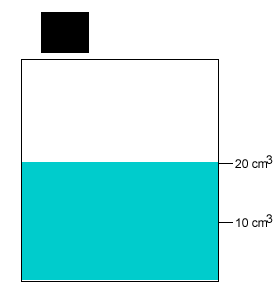A 10 gram salt crystal was dropped into 20cm3 of water. What is the density of the salty water? The density of pure water is 1g/cm3 . The mass of the pure water is 20g. The mass of the salt crystal is 10g. Total mass of the solution is 30g. The total volume is 20cm3 . Density = mass/volume = 30g/20cm3 = 1.5g/cm3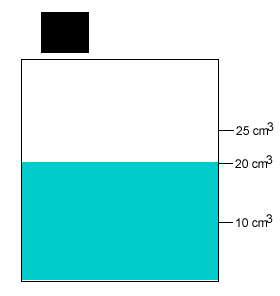A 20 gram salt crystal was dropped into 20cm3 of water. What is the density of the salty water? The density of pure water is 1g/cm3 . The mass of the pure water is 20g. The mass of the salt crystal is 20g. Total mass of the solution is 40g. The total volume is 25cm3 . Density = mass/volume = 40g/25cm3 = 1.6g/cm3 Continue